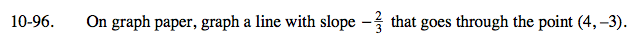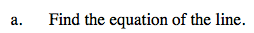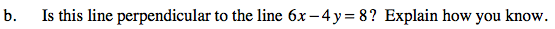### Home > CAAC > Chapter 10 > Lesson 10.2.5 > Problem10-96

10-96.Substitute in the known values for slope x, and y to find the y-intercept of this line.

$-3=4\left(-\frac{2}{3}\right)+b$

Now solve this equation for b.

$-\frac{9}{3}+\frac{8}{3}=b$

$-\frac{1}{3}=b$

$y=-\frac{2}{3}x-\frac{1}{3}$The slope of a line (a) perpendicular to another line (b) is the opposite reciprocal of line (b)'s slope.
Solve this equation for y to find the slope and compare it to the slope of the line in part (a).